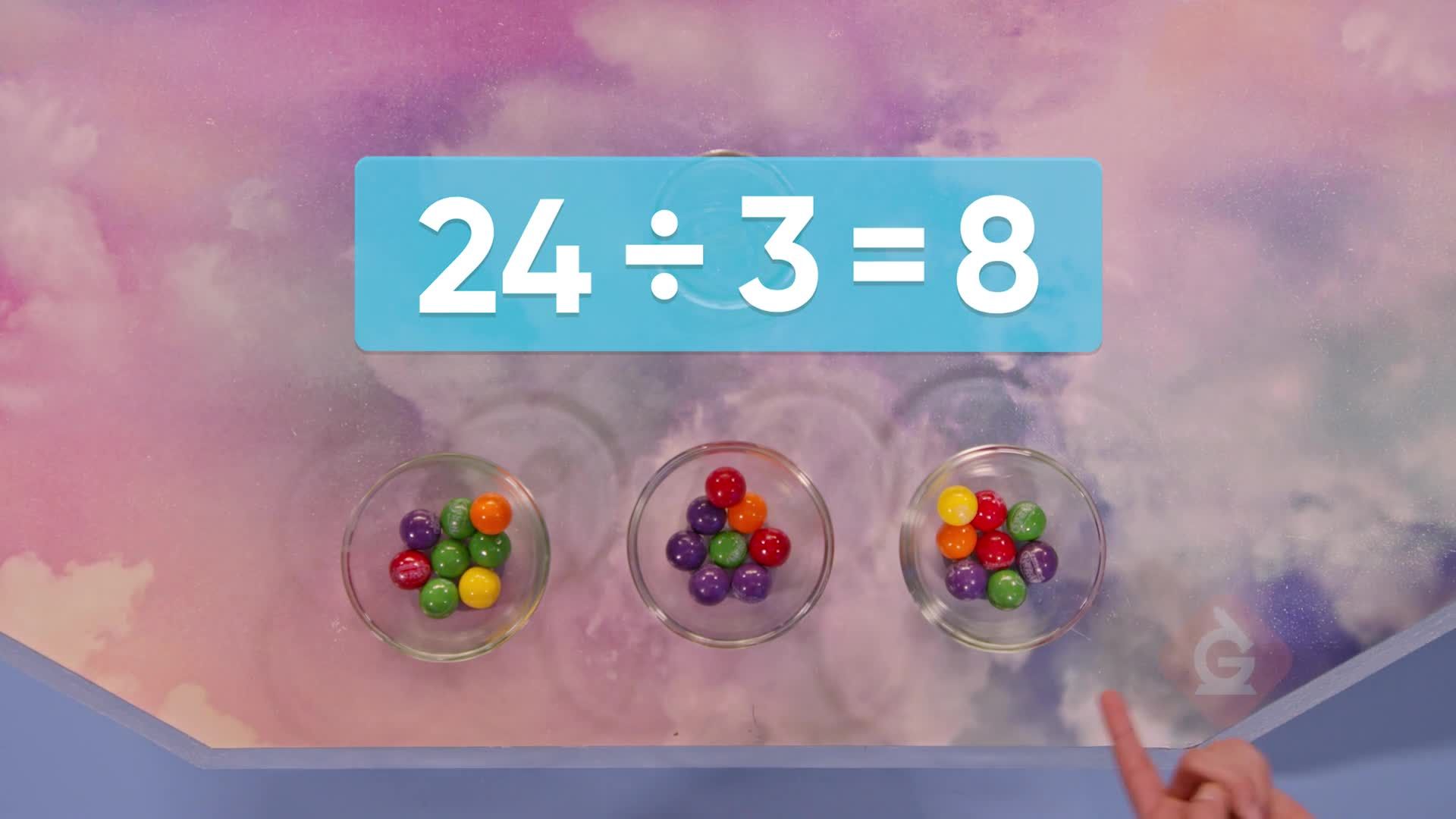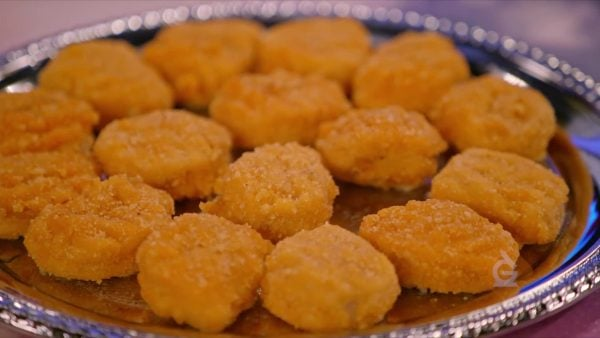Intro to Division Math Video For Kids | 3rd, 4th & 5th Grade
1%
It was processed successfully!WHAT IS INTRO TO DIVISION?

Division is dividing objects into equal groups. Multiplication and division are related operations, like addition and subtraction.

To better understand intro to division…

WHAT IS INTRO TO DIVISION?. Division is dividing objects into equal groups. Multiplication and division are related operations, like addition and subtraction. To better understand intro to division…

## LET’S BREAK IT DOWN!

### CupcakesAdesina, April, and Marcos have 6 cupcakes and they would like to split the cupcakes up equally between the three of them. Marcos suggests that they just put some on every plate, and then add and subtract until they are all equal. April suggests they distribute them one at a time. Both ways could work. They begin by giving each person one cupcake. Then each person gets another. When each person has two cupcakes, there are no more left to distribute. This is called division: When we divide 6 cupcakes into three equal groups, each group has 2 cupcakes. Written as math, this means that 6 divided by 3 is 2. Similarly, we can divide 12 strawberries among the 3 friends. Give each friend 1 strawberry at a time. In the end, each friend has 4 strawberries. There are 3 equal groups with 4 in each group, so 12 divided by 3 equals 4. Now you try: If you divide 10 apples evenly among 5 people, how many apples does each person get?

Cupcakes Adesina, April, and Marcos have 6 cupcakes and they would like to split the cupcakes up equally between the three of them. Marcos suggests that they just put some on every plate, and then add and subtract until they are all equal. April suggests they distribute them one at a time. Both ways could work. They begin by giving each person one cupcake. Then each person gets another. When each person has two cupcakes, there are no more left to distribute. This is called division: When we divide 6 cupcakes into three equal groups, each group has 2 cupcakes. Written as math, this means that 6 divided by 3 is 2. Similarly, we can divide 12 strawberries among the 3 friends. Give each friend 1 strawberry at a time. In the end, each friend has 4 strawberries. There are 3 equal groups with 4 in each group, so 12 divided by 3 equals 4. Now you try: If you divide 10 apples evenly among 5 people, how many apples does each person get?

### Chicken NuggetsAdesina shows April and Marcos how to divide without having to move the actual objects one by one. They have 16 chicken nuggets to split evenly between April and Marcos. Instead of moving actual chicken nuggets, they draw 16 dots to represent the nuggets, and they draw 2 circles to represent the plates. Now they can use the previous method by adding dots to both plates until each person has the same amount, and all the dots are used up. They draw 1 on each plate, then 2 each, 3, 4, 5, 6, 7, and finally, 8 on each plate. Then all the dots are used up. This means that if 16 is divided into two equal groups, each group has 8. A faster way would be to distribute more than one dot at once, still making sure that the same number of dots is distributed each time. If we split up 21 carrots among 3 people, we can try distributing 3 carrots at a time. First each person receives 3 carrots, and then each person receives another 3 carrots. Then 18 carrots have been distributed, and there are 3 left. We split up the remaining 3 one at a time. Then, each person has 7 carrots. That means that 21 divided by 3 is 7. Now you try: How can you divide 14 chocolate almonds evenly among 2 people?

Chicken Nuggets Adesina shows April and Marcos how to divide without having to move the actual objects one by one. They have 16 chicken nuggets to split evenly between April and Marcos. Instead of moving actual chicken nuggets, they draw 16 dots to represent the nuggets, and they draw 2 circles to represent the plates. Now they can use the previous method by adding dots to both plates until each person has the same amount, and all the dots are used up. They draw 1 on each plate, then 2 each, 3, 4, 5, 6, 7, and finally, 8 on each plate. Then all the dots are used up. This means that if 16 is divided into two equal groups, each group has 8. A faster way would be to distribute more than one dot at once, still making sure that the same number of dots is distributed each time. If we split up 21 carrots among 3 people, we can try distributing 3 carrots at a time. First each person receives 3 carrots, and then each person receives another 3 carrots. Then 18 carrots have been distributed, and there are 3 left. We split up the remaining 3 one at a time. Then, each person has 7 carrots. That means that 21 divided by 3 is 7. Now you try: How can you divide 14 chocolate almonds evenly among 2 people?

### PiesThere are 30 pies on a table. The 30 pies need to be divided equally between 10 people. It could be a lot of work if you solve it by counting dots. However, you can use your knowledge of multiplication to help you divide. Since multiplication is adding equal groups, you can think of the problem as a multiplication problem as well. There are 10 groups. How many are in each group if there are 30 in all? 10 × ? = 30. Since 10 groups of 3 equal 30, 30 can be split into 10 equal groups of 3. So, 30 divided by 10 is equal to 3. Multiplication can be thought of as putting groups together, and division can be thought of as splitting groups apart. To solve 32 divided by 8, ask: 8 times what number equals 32? 8 × 4 = 32, so 32 divided by 8 equals 4. Now you try: What is 28 divided by 4?

Pies There are 30 pies on a table. The 30 pies need to be divided equally between 10 people. It could be a lot of work if you solve it by counting dots. However, you can use your knowledge of multiplication to help you divide. Since multiplication is adding equal groups, you can think of the problem as a multiplication problem as well. There are 10 groups. How many are in each group if there are 30 in all? 10 × ? = 30. Since 10 groups of 3 equal 30, 30 can be split into 10 equal groups of 3. So, 30 divided by 10 is equal to 3. Multiplication can be thought of as putting groups together, and division can be thought of as splitting groups apart. To solve 32 divided by 8, ask: 8 times what number equals 32? 8 × 4 = 32, so 32 divided by 8 equals 4. Now you try: What is 28 divided by 4?You’re going on a road trip that is 72 kilometers long. That’s a long trip, so Marcos suggests you split it up into 6 parts. Solve 72 ÷ 6 to find out how long each part will be. 72 ÷ 6 = ? is the same as 6 × ? = 72. You know that 6 × 12 = 72, so each part of the trip will be 12 kilometers long. Now you try: You’re going on a hike that’s 32 kilometers long. You want to do it over 4 days. How far will you hike each day?

Road Trip You’re going on a road trip that is 72 kilometers long. That’s a long trip, so Marcos suggests you split it up into 6 parts. Solve 72 ÷ 6 to find out how long each part will be. 72 ÷ 6 = ? is the same as 6 × ? = 72. You know that 6 × 12 = 72, so each part of the trip will be 12 kilometers long. Now you try: You’re going on a hike that’s 32 kilometers long. You want to do it over 4 days. How far will you hike each day?

## INTRO TO DIVISION VOCABULARY

Equal
Containing the same amount, or the same size.
Multiplication
Division
An operation that helps us to share numbers or objects equally.
Model
A drawing or diagram that helps represent the objects we are counting or doing math with.
Distribute
Share or put in each group.
Inverse operations
Operations that do the opposite of each other.
Put together.
A math sentence that says that two amounts are equal to each other.

## INTRO TO DIVISION DISCUSSION QUESTIONS

### What are some methods you can use to divide 12 candies among 3 people?

I can give one candy to each person, and then another, and then another until they are all gone. I can also give two candies to each person, and then give another 2 to each person. Or I can find the related multiplication equation and solve it that way.

### I have 15 candies that I want to distribute evenly among 3 people. I give each person 3 candies and then there are 6 left. So I give three to the first person, one to the second person, and two to the last person. Now they’re all gone. Did I distribute the candies right?

No, you have to always give the same number of candies to each person, because when we divide, we have to make equal-sized groups.

### I have 16 candies I want to distribute evenly among 2 people. First I give each person 3 candies. Now there are 10 left. I give each person 3 candies again. Now there are 4 left. Can I give each person 3 candies again?

No, there are only 4 candies left. We have to give the same number of candies to each person, so we can give each person 2 more candies. Then each person has 3 + 3 + 2 = 8 candies. Therefore, 16 divided by 2 is 8.

### What is the multiplication sentence that can help you solve 18 ÷ 3?

We have to ask “3 times what is equal to 18?" Since 3 × 6 = 18, 18 ÷ 3 = 6.

### What is the multiplication sentence that can help you solve 54 ÷ 6?

We have to ask “6 times what is equal to 54?" Since 6 × 9 = 54, 54 ÷ 6 = 9.
X

## Success

We’ve sent you an email with instructions how to reset your password.
Ok

## Choose Your Free Trial Period### 7 DaysContinue to Lessons### 90 DaysGet 90 days free by inviting other teachers to try it too.

Share with Teachers

## Get 90 Days Free

### By inviting 4 other teachers to try it too.

4 required

Skip, I will use a 7 days free trial

## Thank You!

Enjoy your free 90 days trial